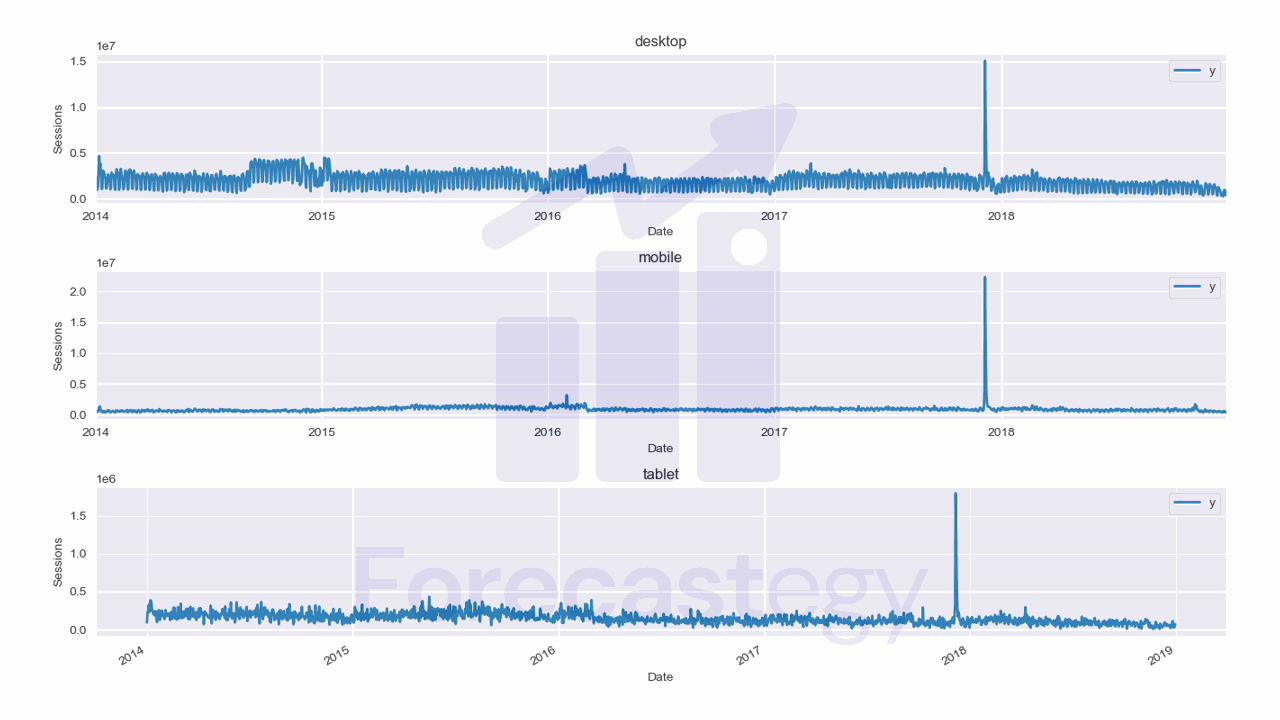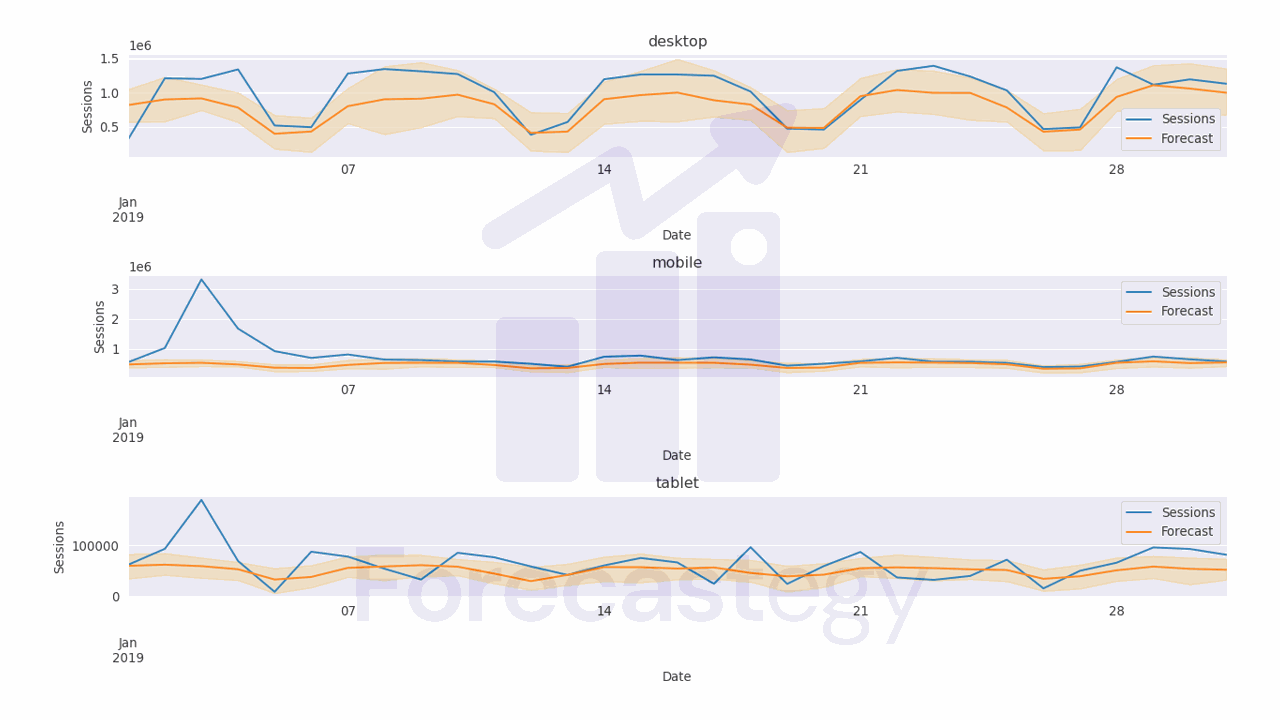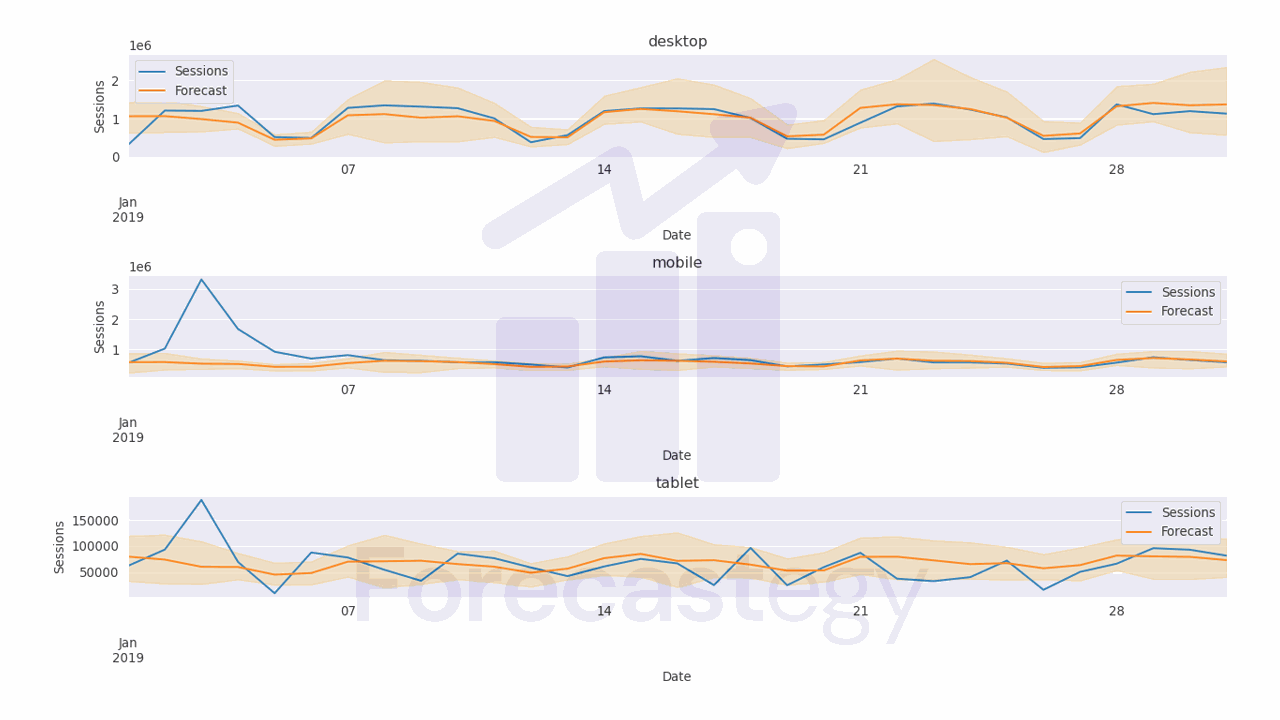In this article, we will explore the use of Gaussian Processes for time series forecasting in Python, specifically using the GluonTS library.

GluonTS is an open-source toolkit for building and evaluating state-of-the-art time series models.

One of the key benefits of using Gaussian Processes for time series forecasting is that they can provide probabilistic predictions.

Instead of just predicting a point estimate for the next value in the time series, GPs can provide a distribution over possible values, allowing us to quantify our uncertainty.

This is not exclusive to GPs, so we have to take into account that it takes more time to train a GP model than a traditional model like ARIMA.

Let’s dive in!

## Installing GluonTS#

Before installing GluonTS, we need to install MXNet. Check out the official installation guide for more details.

Then you can install GluonTS using pip:

``````pip install gluonts
``````

## Preparing The Data For Gaussian Processes In GluonTS#

We will use data from the City of Los Angeles website traffic dataset on Kaggle.

This dataset contains the number of user sessions for the City of Los Angeles website for each day from 2014 to 2019.

Sessions are periods of time when a user is active in a website.

Usually they are measured by looking at user activity and have a timeout after 30 minutes since the last user action.

For example, right now you are in a session on my website. If you leave the page and come back after 2 hours, you will be in a new session.

Let’s load the data and take a look at it:

``````import pandas as pd
import numpy as np
from matplotlib import pyplot as plt
import os

parse_dates=['Date']).loc[:, ['Date', 'Device Category', 'Sessions']]
data = data.groupby(['Date', 'Device Category'], as_index=False)['Sessions'].sum()

fixes = pd.DataFrame({'Date': pd.to_datetime(['2016-12-01', '2018-10-13']),
'Device Category': ['tablet', 'tablet'] ,
'Sessions': [0, 0]})
data = pd.concat([data, fixes], axis=0, ignore_index=True)
data = data.sort_values(['Device Category', 'Date']).groupby('Device Category').apply(lambda x: x.fillna(method='ffill'))
``````
Date Device Category Sessions
2014-01-01 00:00:00 desktop 1032805
2014-01-01 00:00:00 mobile 537573
2014-01-01 00:00:00 tablet 92474
2014-01-02 00:00:00 desktop 2359710
2014-01-02 00:00:00 mobile 607544

We load the data with Pandas and take only 3 columns: the date, the device category, and the number of sessions.

The original data has more detailed information about the sessions, like the browser and number of visitors, but we don’t need it for this tutorial.

We then group the data by date and device category and sum the number of sessions for each group.

Here we have 3 time series: one for desktop, one for mobile, and one for tablet.They are stacked in the long format, which means that each row represents a single observation.

This data has two observations missing for the `tablet` device category, so we add them manually as `np.nan`, then fill with the previous day value.

Now we split the data into training and validation sets with a simple time-based split:

``````train = data.loc[data['Date'] < '2019-01-01']
valid = data.loc[(data['Date'] >= '2019-01-01') & (data['Date'] < '2019-02-01')]
h = valid['Date'].nunique()
``````

`h` is the horizon, the number of time steps we want to forecast into the future. In this case, the number of days in the validation set.

Splitting the data is important because we want to make sure that the model doesn’t overfit to the data it has seen and can’t generalize to the future.

GluonTS requires us to use a specific object for time series data.

We can easily convert our Pandas DataFrame to a GluonTS dataset using the `from_long_dataframe` method:

``````from gluonts.dataset.pandas import PandasDataset
from gluonts.dataset.multivariate_grouper import MultivariateGrouper

unique_series = train['Device Category'].nunique()
train_ds = PandasDataset.from_long_dataframe(train, target='Sessions', item_id='Device Category', timestamp='Date', freq='D')
grouper_train = MultivariateGrouper(max_target_dim=unique_series)
train_ds = grouper_train(train_ds)
``````

This method takes in four arguments:

• `train` is a Pandas DataFrame containing the training data.
• `target` is the name of the target column (the value we want to forecast)
• `item_id` is the name of the column containing unique identifiers for each time series, in case we have multiple like here.
• `timestamp` is the name of the column containing the time stamps for each observation
• `freq` is the frequency of the time series data, in this case daily.

Next, we have the `grouper_train` variable, which is an instance of the `MultivariateGrouper` class.

This class is used to group together time series data for models that implement multivariate forecasting in GluonTS.

We set the `max_target_dim` parameter to 3, which is the number of time series we have.

Finally, we’re passing the `train_ds` variable to the `grouper_train()` method to transform the data based on the criteria we specified earlier.

## Training A Gaussian Process Model For Time Series Forecasting#

We will use a flavor of Gaussian Process called GP-Copula or GPVar.

This model is a mix of a Gaussian Process and a recurrent neural network (usually LSTM).

You can find all the details in GP-Copula’s original paper.

``````from gluonts.mx import Trainer, GPVAREstimator

estimator = GPVAREstimator(freq='D', prediction_length=h, target_dim=unique_series, context_length=30, trainer = Trainer(ctx='gpu', epochs=5, learning_rate=1e-3))
predictor = estimator.train(train_ds)
``````

We instantiate the `GPVAREstimator` class and pass in the following parameters:

• `freq`: Frequency of the time series data. In this example, it’s set to `D` to indicate that the data is daily.
• `prediction_length`: Number of time steps to be predicted by the model.
• `target_dim`: Dimension of the target variable. This is typically the number of series we have. In this case, it’s set to `unique_series`, which is the number of unique device categories in the training data.
• `context_length`: Length of the training context. This is the number of time steps the model uses as inputs.
• `trainer`: An instance of the `Trainer` class which defines how the model is trained. It specifies parameters such as the number of epochs and learning rate. In this example, it’s set to use 5 epochs, a learning rate of 1e-3, and to run on a GPU.

The `learning_rate` is a very important parameters that we will optimize later. It defines the step size of the optimzation algorithm used to train the model.

I found, through experience, that fixing a number of epochs and optimizing the learning rate is a good strategy to find the best model while avoiding overfitting.

If you don’t have a GPU, you can set `ctx='cpu'` to run the model on the CPU.

The predictor object is created by calling the `train` method of the `estimator` object, passing in the training dataset (`train_ds`) as an argument.

Notice that, different than the usual scikit-learn API, the `train` (or `fit`) method returns an object that can be used to generate forecasts.

## Generating Forecasts#

Let’s get the forecasts for the validation set:

``````pred = list(predictor.predict(train_ds))
``````

The `predict` method takes in a dataset and returns a generator object with the forecasts.

In this case it’s a 100 by 31 by 3 array, where 100 is the number of samples it generated from the posterior distribution, 31 is the number of time steps in the validation set (the prediction horizon), and 3 is the number of unique time series.

Let’s process this data into a Pandas DataFrame to evaluate the model:

``````all_preds = list()
item = pred
p = np.median(item.samples, axis=0).clip(0)
p10 = np.percentile(item.samples, 10, axis=0).clip(0)
p90 = np.percentile(item.samples, 90, axis=0).clip(0)
dates = pd.date_range(start=item.start_date.to_timestamp(), periods=len(p), freq='D')

for name, q in [('p50', p), ('p10', p10), ('p90', p90)]:
wide_p = pd.DataFrame({'Date': dates})
for i, device in enumerate(train_['Device Category'].unique()):
wide_p[device] = q[:, i]
wide_p = wide_p.melt(id_vars='Date', var_name='Device Category', value_name=name)
all_preds.append(wide_p.set_index(['Date', 'Device Category']))

all_preds = pd.concat(all_preds, axis=1).reset_index()
all_preds = all_preds.merge(valid, on=['Date', 'Device Category'], how='left')
``````The code above computes the median, 10th percentile, and 90th percentile of the predicted values.

It uses `numpy` to compute these percentiles along the samples axis of the predicted values array.

The for loop then creates a Pandas dataframe with the date range and the predicted values for each device category as the columns.

The `melt` function is used to unpivot the dataframe, converting the device categories to a single column, and the values to a separate column. The resulting dataframe for each percentile is then appended to the `all_preds` list.

After computing the predicted values for each percentile, the code concatenates the dataframes in `all_preds` along the columns axis, resulting in a single dataframe with dates, columns for each percentile (`p50`, `p10`, `p90`) and device category.

Date Device Category p50 p10 p90 Sessions
2019-01-01 00:00:00 desktop 1.06014e+06 625134 1.43136e+06 315674
2019-01-02 00:00:00 desktop 1.06372e+06 643280 1.47342e+06 1209157
2019-01-03 00:00:00 desktop 986666 660598 1.32867e+06 1199978
2019-01-04 00:00:00 desktop 892633 734292 1.14378e+06 1339970
2019-01-05 00:00:00 desktop 445984 282950 591550 516879

We merge the dataframe with the validation data based on the date and device category columns so it’s straightforward to compute the pinball loss.

## Evaluating The Model#

The Pinball loss is a commonly used metric in time series forecasting that measures the deviation of the predicted quantiles from the actual values at a specific quantile level.

``````from sklearn.metrics import mean_pinball_loss
for q in [0.1, 0.5, 0.9]:
print(f'pinball loss at {q}: {mean_pinball_loss(all_preds["Sessions"], all_preds[f"p{int(q*100)}"], alpha=q)}')
``````

The code imports the `mean_pinball_loss` function from scikit-learn.

It then calculates the pinball loss for quantiles of 0.1, 0.5, and 0.9 using a for loop.

Inside the loop, the function is called with the following arguments: the actual values of sessions (`all_preds["Sessions"]`), the predicted values for the corresponding quantile (`all_preds[f"p{int(q*100)}"]`), and the value of `alpha` (which is the quantile).

The final numbers are:

• pinball loss at 0.1: 33952.08
• pinball loss at 0.5: 87465.95
• pinball loss at 0.9: 68380.55

Let’s see if we can improve the model by optimizing the hyperparameters.

## Optimizing The Hyperparameters Of The Gaussian Process With Optuna#

We will use Optuna to optimize the hyperparameters of the Gaussian Process model.

This is a very simple library that uses Tree-structured Parzen Estimator (TPE) to optimize the hyperparameters of the model.

We just need to encapsulate the code that trains the model in a function that takes a `trial` object as an argument.

The `trial` object is a single step of the optimization process.

The function returns a list of values, as we want to optimize the pinball loss at quantiles of 0.1, 0.5, and 0.9.

``````def objective(trial):
context_length = trial.suggest_int('context_length', 7, 400)
learning_rate = trial.suggest_loguniform('learning_rate', 1e-5, 1e-1)
num_layers = trial.suggest_int('num_layers', 1, 4)
num_cells = trial.suggest_int('num_cells', 8, 128)
cell_type = trial.suggest_categorical('cell_type', ['lstm', 'gru'])
dropout_rate = trial.suggest_uniform('dropout_rate', 0.1, 0.9)
rank = trial.suggest_int('rank', 1, 3)

estimator = GPVAREstimator(freq='D', prediction_length=h, target_dim=unique_series,
context_length=context_length, num_layers=num_layers,
num_cells=num_cells, cell_type=cell_type, dropout_rate=dropout_rate,
rank=rank,  trainer = Trainer(ctx='gpu', epochs=5, learning_rate=learning_rate))
predictor = estimator.train(train_ds)
pred = list(predictor.predict(train_ds))

all_preds = list()
item = pred
p = np.median(item.samples, axis=0).clip(0)
p10 = np.percentile(item.samples, 10, axis=0).clip(0)
p90 = np.percentile(item.samples, 90, axis=0).clip(0)
dates = pd.date_range(start=item.start_date.to_timestamp(), periods=len(p), freq='D')
for name, q in [('p50', p), ('p10', p10), ('p90', p90)]:
wide_p = pd.DataFrame({'Date': dates})
for i, device in enumerate(train_['Device Category'].unique()):
wide_p[device] = q[:, i]
wide_p = wide_p.melt(id_vars='Date', var_name='Device Category', value_name=name)
all_preds.append(wide_p.set_index(['Date', 'Device Category']))

all_preds = pd.concat(all_preds, axis=1).reset_index()
all_preds = all_preds.merge(valid, on=['Date', 'Device Category'], how='left')

return [mean_pinball_loss(all_preds["Sessions"], all_preds[f"p{int(q*100)}"], alpha=q) for q in [0.1, 0.5, 0.9]]
``````

We define the hyperparameters search space using the `suggest_*` methods of the `trial` object.

These methods will sample a value from the specified range or distribution.

Here is a breakdown of each parameter:

• `context_length`: the number of time steps in the past that the model should use to make a prediction about the future. I set a range from 7 to 400 so we can test very short and very long windows.
• `learning_rate`: the step size at which the model adjusts its weights during training. A small learning rate can lead to slower convergence, while a large learning rate can lead to overshooting the optimal weights. A good rule of thumb is to use a log-uniform distribution between 1e-5 and 1e-1.
• `num_layers`: the number of layers in the RNN model. A deeper model can capture more complex patterns in the data, but can also be prone to overfitting. Let’s try values between 1 and 4. More layers than that are usually not necessary for time series forecasting.
• `num_cells`: the number of units or neurons in each layer of the model. A number between 8 and 128 should be enough.
• `cell_type`: the type of recurrent neural network (RNN) cell to use in the model. This can be either Long Short-Term Memory (LSTM) or Gated Recurrent Unit (GRU). These are both popular choices for time series forecasting.
• `dropout_rate`: the rate at which to randomly drop out units in the model during training to prevent overfitting. A good range is between 0.1 and 0.9.
• `rank`: the rank of the distribution that will be used to sample the predictions. Default is 2, but let’s try values between 1 and 3.

The rest of the code is similar to the previous example.

To run the optimization, we just need to call the `optimize` method of the `study` object.

``````import optuna
study = optuna.create_study(directions=['minimize', 'minimize', 'minimize'])
study.optimize(objective, n_trials=20, timeout=900)
``````

We set the `directions` of each returned value to `minimize` because we want to minimize the pinball losses at all quantiles.

`n_trials` is the number of steps to run. 20 is usually a good number to start with. 30 is enough for most cases.

The `timeout` argument is the maximum time in seconds to run the optimization.The attribute `study.best_trials` returns a list of the best trials, meaning the trials that returned the lowest values for any of the objectives.

After inspecting it, I found the second element of the returned list to be the minimum for 2 of the 3 objectives, so I picked it.

``````best_trial = study.best_trials
best_trial.params
{'context_length': 270,
'learning_rate': 0.013101512029143874,
'num_layers': 1,
'num_cells': 84,
'cell_type': 'lstm',
'dropout_rate': 0.16228795203947746,
'rank': 2}
``````

To get the corresponding values for the objectives, we can call the `values` attribute of the `best_trial` object.

``````best_trial.values
[31605.068581359206, 64329.63781081989, 69049.05124747986]
``````

The model improved, but I found it takes too long to reach a good solution compared to alternatives like CNNs, “pure” LSTMs or Kalman filters